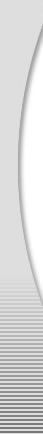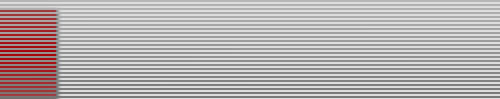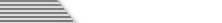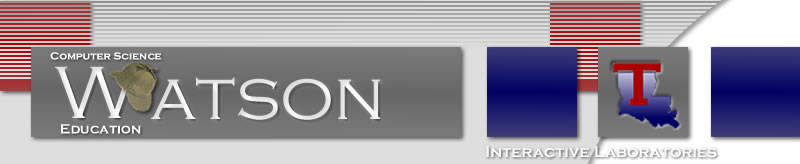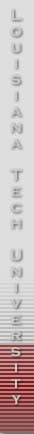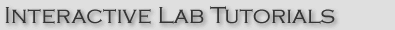The Watson lab tutorials provide easy to understand, visually attractive, interactive walkthroughs of the Watson labs. The presentation and structure of the tutorials generally mirror the organization of the User Manual. Thus, both provide complementary mechanisms for acquiring familiarity with Watson.

The following tutorials are available:

• General Watson Tutorial
• Database Tutorial
• Data Structures Tutorial
• Graphics Tutorial
• JavaScript Tutorial
• Object-Oriented Tutorial
• Assembly Tutorial
• Digital Logic TutorialThe Watson Standard Features Tutorial provides a step by step walkthrough explaining the general features common to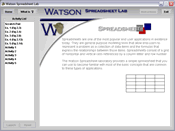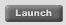all Watson Labs. Section one takes the user through choosing and starting an activity. Section two shows the user how to access the help function and get descriptions for any Watson feature.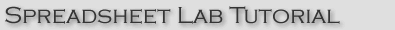The Watson Spreadsheet Lab Tutorial provides a step by step walkthrough explaining the functions and tools of typical spreadsheet operations in four sections. Section one concerns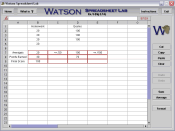the adding the contents of cells; section two deals with averaging the contents of cells; section three deals with decimal formatting of selected cells; and section four deals with the particulars of cell reference and duplication.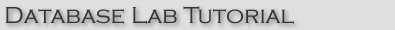The Watson Database Lab Tutorial provides a step by step walkthrough showing the operations of database queries using relational expressions in three sections. Section one concernsthe process of projecting a relation; section two deals with the process of joining two or more relations; and section three deals with the process of deleting relations.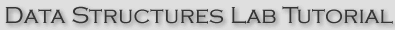The first tutorial introduces the user to a stack. It covers pushing an item on the stack and popping an item off the stack. The second tutorial introduces the user to a queue. It covers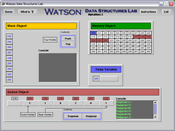enqueuing an item into a queue. Also dequeuing an item is covered in this tutorial. The third tutorial covers how memory is used by a queue and stack. The queue moves through memory similar to a snake while a stack remains in the same place shrinking and growing.The Watson Graphics Lab Tutorial provides a step by step walkthrough explaining the lab features and the code used to create graphic primatives. Section one details drawinga graphic primative in interactive or free-hand mode; section two deals with drawing a primative using code-based generation.The javascript tutorial will aid the user in understanding the core concepts presented in Watson's Javascript Lab. The first tutorial will lead the user through the steps necessary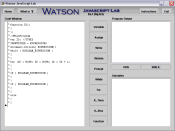to create functions and function calls. A second tutorial will allow the user to create a basic while loop and run the created code.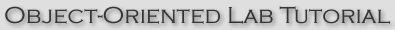The Object-Oriented tutorial provides the user with an understanding of the basic functionality of the Object-Oriented Lab and it's associated concepts. The first tutorial creates an instanceof a user created object. The second tutorial explains how to edit and add methods. Lastly,there is a lesson on inheritance.The Watson Assembly Lab Tutorial provides a step by step walkthrough showing the operations, functions and syntax of common assembly language programming in six sections. Section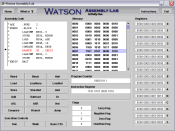one concerns the use of labels; Section two deals with the registers; Section three illustrates assembly binary addition; Section four concerns the arithmatic shift operation; Section five deals with the basic logical operations; and section six explains how to execute an assembly program.The first tutotorial covers creating gates. The second tutorial shows a user how to delete gates. The third tutorial shows how to power the circuit. The fourth tutorial goes over the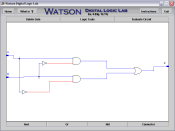logic tools.